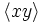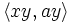# Central product of D8 and Z4

View a complete list of particular groups (this is a very huge list!)[SHOW MORE]

## Definition

This group of order 16 is defined in the following equivalent ways:

1. It is the central product of the dihedral group of order eight and cyclic group of order four over a common cyclic central subgroup of order two.
2. It is the central product of the quaternion group and cyclic group of order four over a common cyclic central subgroup of order two.

It is given by the presentation:$G := \langle a,x,y \mid a^4 = y^4 = x^2 = e, a^2 = y^2, xax = a^{-1}, ay = ya, xy = yx \rangle$.

Here,$\langle a,x \rangle$ is the dihedral group of order eight and$\langle y \rangle$ is the cyclic group of order four.

## Group properties

Property Satisfied Explanation Comment
Abelian group No$a$ and$x$ don't commute
Nilpotent group Yes Prime power order implies nilpotent
UL-equivalent group No Nilpotency class two, but center does not coincide with derived subgroup. See nilpotent not implies UL-equivalent
Metacyclic group No
Supersolvable group Yes
Solvable group Yes Nilpotent implies solvable
T-group No$\langle a^4,x \rangle \triangleleft \langle a^2,x \rangle$, which is normal, but$\langle a^4, x \rangle$ is not normal
Monolithic group Yes Unique minimal normal subgroup of order two
One-headed group No Seven distinct maximal normal subgroups of order eight
Directly indecomposable group Yes
Centrally indecomposable group No
Splitting-simple group No

## Subgroups

Further information: Subgroup structure of central product of D8 and Z4

1. The trivial subgroup. Isomorphic to trivial group. (1)
2. The subgroup$\langle a^2 \rangle$. This is the unique normal subgroup of order two, and is contained in the center. Isomorphic to cyclic group:Z2. (1)
3. The subgroups$\langle x \rangle$,$\langle ax \rangle$,$\langle a^2x \rangle$,$\langle a^3x \rangle$. These come in two conjugacy classes of 2-subnormal subgroups, one comprising$\langle x \rangle$ and$\langle a^2x \rangle$ and the other comprising$\langle ax \rangle$ and$\langle a^3x \rangle$. However, they are all automorphic subgroups. Isomorphic to cyclic group:Z2. (4)
4. The subgroups$\langle ay \rangle$ and$\langle a^3y \rangle$. These form a single conjugacy class of 2-subnormal subgroups. Isomorphic to cyclic group:Z2. (2)
5. The subgroup$\langle y \rangle$ of order four. This is the center. Isomorphic to cyclic group:Z4. (1)
6. The subgroups$\langle a \rangle$,$\langle xy \rangle$ and$\langle axy \rangle$. These are normal subgroups but are automorphic subgroups: they are related by outer automorphisms. Isomorphic to cyclic group:Z4. (3)
7. The subgroup$\langle a^2, x \rangle$,$\langle a^2, ax \rangle$ and$\langle a^2, ay \rangle$. These are all normal subgroups but are related by outer automorphisms. Isomorphic to Klein four-group. (3)
8. The subgroup$\langle a, xy \rangle$. This is an isomorph-free subgroup of order eight, containing the three non-characteristic cyclic subgroups of order four. Isomorphic to quaternion group. (3)
9. The subgroups$\langle a,y \rangle$,$\langle x, y \rangle$ and$\langle ax, y \rangle$. These are all normal and related by outer automorphisms. Isomorphic to direct product of Z4 and Z2. (3)
10. The subgroups$\langle a,x \rangle$,$\langle xy, ay \rangle$ and$\langle axy, ay \rangle$. These are all normal and are related by outer automorphisms. Isomorphic to dihedral group:D8. (3)
11. The whole group. (1)

## GAP implementation

### Group ID

This finite group has order 16 and has ID 13 among the groups of order 16 in GAP's SmallGroup library. For context, there are 14 groups of order 16. It can thus be defined using GAP's SmallGroup function as:

SmallGroup(16,13)

For instance, we can use the following assignment in GAP to create the group and name it$G$:

gap> G := SmallGroup(16,13);

Conversely, to check whether a given group$G$ is in fact the group we want, we can use GAP's IdGroup function:

IdGroup(G) = [16,13]

or just do:

IdGroup(G)

to have GAP output the group ID, that we can then compare to what we want.